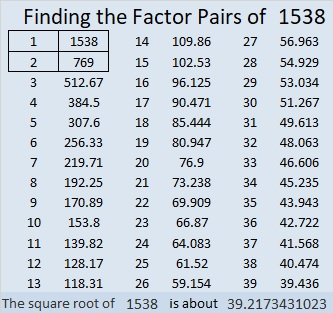# 1538 Second Letter of My Message

### Today’s Puzzle:

This puzzle is the second letter in a message I want to give you. I will give more letters in a few days. How many letters will you need to figure out the message?

I gave you the clues of this level 3 multiplication table puzzle in an order that makes finding the solution easier. It can be solved by finding the common factor of the two clues in the first row of the puzzle and then working your way down the puzzle row by row until you have found all the factors from 1 to 12 for both the first column and the top row.### Factors of 1538:

• 1538 is a composite number.
• Prime factorization: 1538 = 2 × 769.
• 1538 has no exponents greater than 1 in its prime factorization, so √1538 cannot be simplified.
• The exponents in the prime factorization are 1 and 1. Adding one to each exponent and multiplying we get (1 + 1)(1 + 1) = 2 × 2 = 4. Therefore 1538 has exactly 4 factors.
• The factors of 1538 are outlined with their factor pair partners in the graphic below.### More about the Number 1538:

1538 is the sum of two squares:
37² + 13² = 1538.

1538 is the hypotenuse of a Pythagorean triple:
962-1200-1538 calculated from 2(37)(13), 37² – 13², 37² + 13².